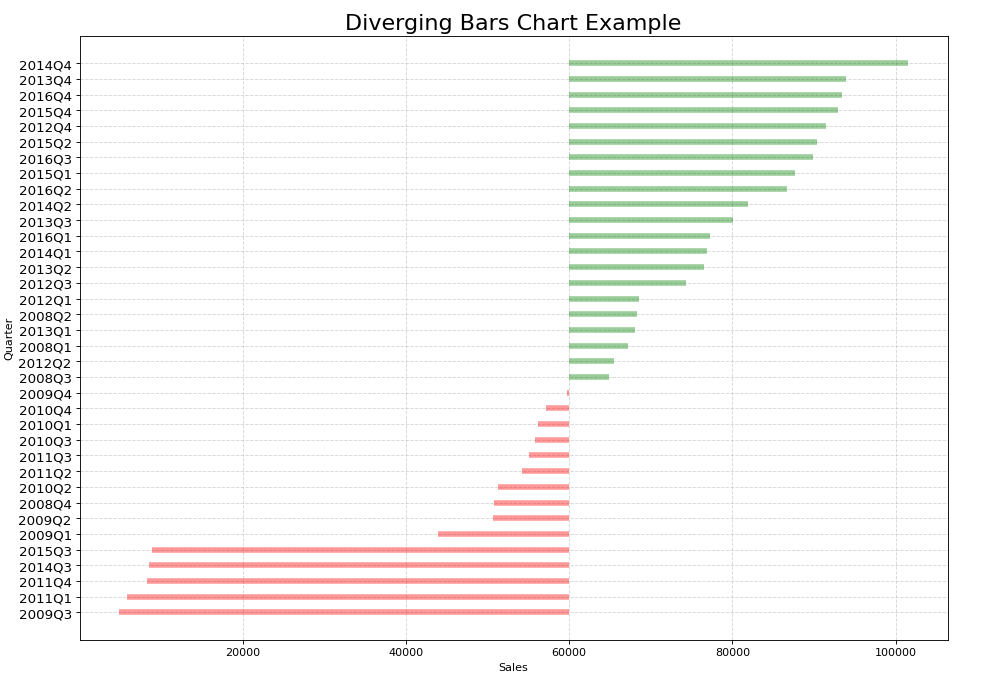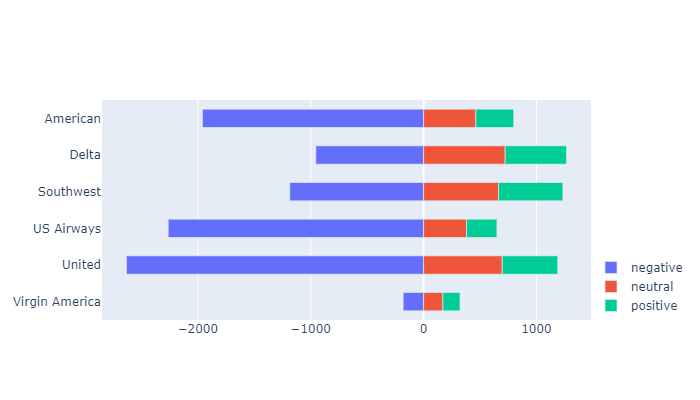Skip to content
Related Articles
Diverging Bar Chart using Python
• Last Updated : 15 Mar, 2021

Diverging Bar Charts are used to ease the comparison of multiple groups. Its design allows us to compare numerical values in various groups. It also helps us to quickly visualize the favorable and unfavorable or positive and negative responses. The bar chart consisted of a combination of two horizontal bars starting from the middle- one bar extends out from right to left and the other extends out from left to right. The length of the bar is corresponding to the numerical value it represents.

Commonly the two diverging bars are represented with different colors. Values to the left are usually but not necessarily negative or unsatisfactory responses.

Python doesn’t have a particular function to plot diverging bar charts. The alternate way is to use hlines function to draw horizontal lines with a certain value of linewidth to represent them as horizontal bars.

Datasets in use:

### Method 1: Using Matplotlib

Approach :

• Import module
• Import or create data
• Preprocess the dataset and clean out the unnecessary noise
• Specify the colors for representing the horizontal bars
• Sort the values in ascending order
• Set the labels for the x-axis and y-axis as well as the title of the chart
• Display the Diverging Bar Chart

Example 1:

## Python

 `import` `pandas as pd``import` `matplotlib.pyplot as plt``import` `string as ``str`` ` ` ` `# Creating a DataFrame from the CSV Dataset``df ``=` `pd.read_csv(``"car_sales.csv"``, sep``=``';'``)`` ` `# Separating the Date and Mercedes-Benz Cars unit sales (USA)``df[``'car_sales_z'``] ``=` `df.loc[:, [``'Mercedes-Benz Cars unit sales (USA)'``]]``df[``'car_sales_z'``] ``=` `df[``'car_sales_z'``] .``str``.replace(``    ``','``, '').astype(``float``)`` ` `# Removing null value``df.drop(df.tail(``1``).index, inplace``=``True``)`` ` `for` `i ``in` `range``(``35``):``    ``# Colour of bar chart is set to red if the sales ``    ``# is < 60000 and green otherwise``    ``df[``'colors'``] ``=` `[``'red'` `if` `float``(``        ``x) < ``60000` `else` `'green'` `for` `x ``in` `df[``'car_sales_z'``]]`` ` `# Sort values from lowest to highest``df.sort_values(``'car_sales_z'``, inplace``=``True``)`` ` `# Resets initial index in Dataframe to None``df.reset_index(inplace``=``True``)`` ` `# Draw plot``plt.figure(figsize``=``(``14``, ``10``), dpi``=``80``)`` ` `# Plotting the horizontal lines``plt.hlines(y``=``df.index, xmin``=``60000``, xmax``=``df.car_sales_z,``           ``color``=``df.colors, alpha``=``0.4``, linewidth``=``5``)`` ` `# Decorations``# Setting the labels of x-axis and y-axis``plt.gca().``set``(ylabel``=``'Quarter'``, xlabel``=``'Sales'``)`` ` `# Setting Date to y-axis``plt.yticks(df.index, df.Date, fontsize``=``12``)`` ` `# Title of Bar Chart``plt.title(``'Diverging Bars Chart Example'``, fontdict``=``{``          ``'size'``: ``20``})`` ` `# Optional grid layout``plt.grid(linestyle``=``'--'``, alpha``=``0.5``)`` ` `# Displaying the Diverging Bar Chart``plt.show()`

Output:### Method 2: Using Plotly

Approach:

• Import required libraries
• Create or import data
• Preprocess the Dataset and clean out the unnecessary noise
• Plot the graph using plotly.graph_objects
• Set the labels for the x-axis and y-axis as well as the legend
• Display the Diverging Bar Chart

Example:

## Python

 `import` `pandas as pd``import` `plotly.graph_objects as go`` ` ` ` `df ``=` `pd.read_csv(``"Tweets.csv"``)``df.head()`` ` `# Preprocessing the dataset to extract only``# the necessary columns``categories ``=` `[``    ``'negative'``,``    ``'neutral'``,``    ``'positive'``]`` ` `# Construct a pivot table with the column``# 'airline' as the index and the sentiments``# as the columns``gfg ``=` `pd.pivot_table(``    ``df,``    ``index``=``'airline'``,``    ``columns``=``'airline_sentiment'``,``    ``values``=``'tweet_id'``,``    ``aggfunc``=``'count'``)`` ` `# Include the sentiments - negative, neutral``# and positive``gfg ``=` `gfg[categories]`` ` `# Representing negative sentiment with negative``# numbers``gfg.negative ``=` `gfg.negative ``*` `-``1`` ` `df ``=` `gfg`` ` `# Creating a Figure``Diverging ``=` `go.Figure()`` ` `# Iterating over the columns``for` `col ``in` `df.columns[``4``:]:`` ` `    ``# Adding a trace and specifying the parameters``    ``# for negative sentiment``    ``Diverging.add_trace(go.Bar(x``=``-``df[col].values,``                               ``y``=``df.index,``                               ``orientation``=``'h'``,``                               ``name``=``col,``                               ``customdata``=``df[col],``                               ``hovertemplate``=``"%{y}: %{customdata}"``))``for` `col ``in` `df.columns:`` ` `    ``# Adding a trace and specifying the parameters``    ``# for positive and neutral sentiment``    ``Diverging.add_trace(go.Bar(x``=``df[col],``                               ``y``=``df.index,``                               ``orientation``=``'h'``,``                               ``name``=``col,``                               ``hovertemplate``=``"%{y}: %{x}"``))`` ` `# Specifying the layout of the plot``Diverging.update_layout(barmode``=``'relative'``,``                        ``height``=``400``,``                        ``width``=``700``,``                        ``yaxis_autorange``=``'reversed'``,``                        ``bargap``=``0.5``,``                        ``legend_orientation``=``'v'``,``                        ``legend_x``=``1``, legend_y``=``0``                        ``)``Diverging`

Output:Attention geek! Strengthen your foundations with the Python Programming Foundation Course and learn the basics.

To begin with, your interview preparations Enhance your Data Structures concepts with the Python DS Course. And to begin with your Machine Learning Journey, join the Machine Learning – Basic Level Course

My Personal Notes arrow_drop_up